## General Question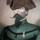# A couple questions about gravity.

Asked by Nullo (21944) August 1st, 2010

1) Earth’s gravity peters out as you move away from it. But is its strength at all affected by how far you travel below the surface?

2) Assume a sphere with the same mass as the Earth. Now assume a hole running from the surface at a given point all the way through to the other side.
If one were to drop a ball into one end of the tunnel, what would happen to it?

Observing members: 0Composing members: 0## 20 Answers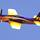1. If you go to the exact center of the earth you will be weightless as the mass of the Earth will be equal on all sides.

2. This is a question is simple oscillation. The ball will oscillate from one side to another, slowly losing energy and then ultimately rest in the middle. Think of a pendulum on a grandfather clock.

Rarebear (25154)“Great Answer” (6) Flag as…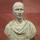@Rarebear answered both points well. However it is important to note that in question two, the ball would oscillate forever if the tunnel was in a vacuum. It would only slow down and come to rest in the middle under the influence of air resistance.

FireMadeFlesh (16558)“Great Answer” (3) Flag as…@FireMadeFlesh A quibble. Nothing can oscillate forever—that’s a perpetual motion machine. There will be some energy loss from heat disappation, etc, and it would, ultimately, stop. But yes, if there is a vacuum, and you assume no energy loss from anything, then yes, it would oscillate forever.

Rarebear (25154)“Great Answer” (1) Flag as…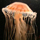@Rarebear
Theoretically being weight less in the centre of the earth is suppose to be the case but as the earth has denser areas and isn’t a perfect sphere in real terms the gravity will be weak but you will likely still get pulled to one side.

Taking a theoretical view here when falling down the hole the gravitation forces to the left and right will cancel each other out so you may not necessarily hit the sides. If we are assuming it’s a vacuum you will go right through to the point the hole ends at and this will be repeated until something stops you.

Remember my last paragraph is theoretical and due to other factors it will not be exactly like this.

dotlin (419)“Great Answer” (1) Flag as…@Rarebear And if you stop, say, ¾ of the way down?

Nullo (21944)“Great Answer” (1) Flag as…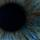@Nullo – Sure; density of the earth is not increasing linearly from top to bottom. I think your ideal is that at some point the amount of mass “under” the ball (towards the center of the earth) becomes vastly outweighed by the huge amount of mass above it in the hole. The problem is that there is all that mass on the other side of middle. So the ball would still resolve somewhere near the center of the earth. (Not exact physical middle – like a pendulum – maybe like magnetic North vs. truth North – possibly within 5% of the absolute center.)

ipso (4476)“Great Answer” (1) Flag as…@dotlin The question says “a sphere with the same mass as the Earth,” without mentioning the distribution of the densities.

FireMadeFlesh (16558)“Great Answer” (0) Flag as…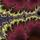This is somewhat similar to Hooke’s 42-minute gravity train. http://en.wikipedia.org/wiki/Gravity_train

Regardless of the distribution of densities of any body, as long as the planet stays fixed, air resistance is disregarded, and the tunnel allows the ball to “fall” without hitting any of the sides, the ball will continue oscillating to two specific points indefinitely.

Vortico (3123)“Great Answer” (0) Flag as…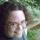Mine is a totally uninformed guess, but for the ball to oscillate like a pendulum, would it not have to be dropped, race to the center and go past, and then slow down as it reaches the surface on the opposite side? This is what I’m wondering: would the acceleration that the ball develops from being dropped in the hole and racing thus for such a distance be enough to send it out of the hole on the opposite side with something like escape velocity? If so, chances are slim that if it “falls back” to the center, that it would land right in the same hole. It would probably be subject to atmospheric disturbance, which would increase with the distance it travels away from the surface on the other side.

This is not my area of expertise, though, so I could be very wrong.

laureth (27163)“Great Answer” (1) Flag as…@dotlin Sure, the Earth isn’t uniform. But it’s also impossible to drill a hole through it too.

@nullo If you stopped ¾ of the way down, you’d have to add up the vectors in the sphere around you. How much mass is above you, how much mass is to the sides, how much mass is to the bottom, etc. You add up the all the gravitational vectors and that will determine your weight. (Your mass, of course, stays the same).

Rarebear (25154)“Great Answer” (0) Flag as…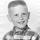You couldn’t have a body with Earth-mass with a hole through it. The gravity from such a body would collapse the tunnel immediately.

AstroChuck (37368)“Great Answer” (1) Flag as…@AstroChuck It’s a theoretical question, and a good one, at that.

Rarebear (25154)“Great Answer” (0) Flag as…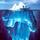This is a classic freshman physics thought problem. Assuming spherical symmetry, including uniform density, the gravitational force acting on a body inside a shaft drilled through the Earth’s center depends only on the mass of the inner sphere below the body at a given depth, with zero contribution from the spherical shell of mass above the body at that depth.

When you work it out, it turns out that the force of attraction decreases linearly from surface to center. This makes the system mathematically equivalent to a body hanging from a spring according to Hooke’s Law. It will oscillate with simple harmonic motion (displacement vs. time forming a perfect sine wave) ignoring air resistance & other friction. If you allow friction, the harmonic motion will dampen until the body comes to rest in equilibrium at the center.

If you fell into the hole at the surface, you would speed up until you raced past the center, then slow down until you stopped just at the surface at the other end of the shaft. Then the process would repeat indefinitely in the absence of friction.

Note that the linear attractive force with distance from the center contrasts with the inverse-square law above the surface.

This was already answered correctly by others, but I wanted to help clarify.

gasman (11296)“Great Answer” (3) Flag as…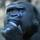Great Question, lots of great answers.

@gasman ”...it turns out that the force of attraction decreases linearly…”

I thought that the gravitational force between objects was exponential with respect to distance (i.e. as objects get closer together the gravitational force between them increases exponentially). Is this a different case since one object is penetrating the other?

gorillapaws (23119)“Great Answer” (0) Flag as…The attractive force between two bodies (earth & moon, say) increases as the inverse square of the distance between their centers (1 / r^2) where r is the distance. This is most definitely NOT an exponential, which would take the form e^(-r) . The confusion perhaps lies in the similarity of decreasing, concave upward curves when graphed. Mathematically, no relation.

gasman (11296)“Great Answer” (0) Flag as…@gasman thanks for clarifying. 1/R^2 certainly isn’t linear though. So why does it behave linearly once one object penetrates the other? Also, what if there was in incredibly small, dense sphere that had a greater mass than a rather large, less-dense sphere with a shaft. Does that change the dynamics at all? Would the large sphere oscillate around the tiny, dense sphere?

gorillapaws (23119)“Great Answer” (0) Flag as…@gorillapaws: The difference is that the deeper you go into the earth, and the closer you get to the center, the less mass there is beneath you to pull you in. At the center there is no mass beneath you and you are weightless. This is quite different than approaching the earth from the outside, where the same total mass is pulling on you at closer & closer distances.

If you could shrink the entire mass of the earth down to the size of marble, then you are always “outside” the earth and indeed 1/r^2 applies as usual. In reality this is similar to neutron stars.

gasman (11296)“Great Answer” (2) Flag as…@gasman GA thanks.

gorillapaws (23119)“Great Answer” (0) Flag as…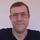If you drop a spoonful of neutron matter onto Earth, the same oscillation would happen. The neutron matter would create the hole on the fly. And the oscillation would slow down over time because of the attrition.

mattbrowne (31595)“Great Answer” (2) Flag as…@mattbrowne I’ll get right on it, then. XD

Nullo (21944)“Great Answer” (2) Flag as…## Answer this questionor

#### Join

to answer.

This question is in the General Section. Responses must be helpful and on-topic.

Your answer will be saved while you login or join.
Have a question? Ask Fluther!
What do you know more about?
or
Knowledge Networking @ Fluther